Ask your WordPress questions! Pay money and get answers fast!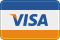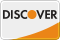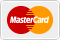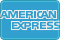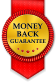# Need an if statement to sort portfolio WordPress

• SOLVED

Hi!

My new site contains several portfolios using the same template block. The portfolios are sorted according to a menu (in WP backend) but I need one of them to be sorted chronologically (by post date), so I'm assuming an if statement would do the trick.

Here's the code in the template -
see at the beginning, it says:

I need this to say instead:

if portfolio = category "writing" (and its children), then order chronologically. Else, order by menu.

The "writing" category has ID 11.

(Or if it makes any difference, you can also make the if statement by the page that portfolio is displayed on - I don't know which one would make the most sense.)

```<!--Output Portfolio items--> <ul class='portfolio-grid row-fluid <?php echo \$lazyload_class; ?>'> <?php \$type = 'portfolio'; \$args=array( 'post_type' => \$type, 'posts_per_page' => \$itemno, 'orderby' => 'menu_order', 'order' => 'ASC', ); if(!empty(\$types)) { \$args['tax_query'] = array( array( 'taxonomy' => 'type', 'field' => 'id', 'terms' => \$types ) ); } global \$post; \$query = new WP_Query( \$args ); \$counter = 1; if(\$query->have_posts()) : while ( \$query->have_posts() ) : \$query->the_post(); // taxonomy terms slugs for isotope filtering \$terms = get_the_terms( \$post->ID, 'type' ); \$term_list = ''; if( is_array(\$terms) ) { foreach( \$terms as \$term ) { \$term_list .= \$term->slug; \$term_list .= ' '; } } // taxonomy terms names for output on hover \$terms = get_the_terms( \$post->ID, 'type' ); \$term_names = ''; if( is_array(\$terms) ) { \$last_elem = end(\$terms); foreach( \$terms as \$term ) { \$term_names .= \$term->name; if (\$term != \$last_elem) { \$term_names .= ', '; } } } // Setting variable width on every 4th item and implementing grayscale option if (\$counter % 4 == 0) { \$size = "width2"; } else { \$size = ""; } if (\$gray == 1) { \$grayscale = "grayscale"; } else { \$grayscale = ""; } // Setting nonce for AJAX security \$postid = \$post->ID; \$nonce = wp_create_nonce('portfolio'); ?> ```

Thank you so much if you can help me! Let me know if you need access to the backend or template files.

2013-08-17

You can add this before the \$type = 'portfolio' line
``` \$cats_array = array(); \$cats_array[] = 11; \$cat_childs = get_categories( array( 'child_of' => 11 ) ); foreach ( \$cat_childs as \$cat_child ) { \$cats_array[] = \$cat_child->term_id; } if ( in_array( get_query_var( 'cat' ), \$cats_array ) ) { \$orderby = 'date'; } else { \$orderby = 'menu_order'; } ```

and change the line 'orderby' => 'menu_order', by 'orderby' => \$orderby

This worked - perfect, thank you!

2013-08-17

```<!--Output Portfolio items--> <ul class='portfolio-grid row-fluid <?php echo \$lazyload_class; ?>'> <?php //code begain \$orderby = 'menu_order'; //default \$current_term = get_queried_object();//get current term if( term_exists( \$current_term->term_id, 'type' ) ){ if(\$current_term->term_id==11){ //if term_id is 11 \$orderby = 'date'; }else{ \$termchildren = get_term_children( \$current_term->term_id, 'type' );//get child term foreach( \$termchildren as \$term){ if( \$term->term_id==\$current_term->term_id ) //if term is child \$orderby = 'date'; break; } } } //end \$type = 'portfolio'; \$args=array( 'post_type' => \$type, 'posts_per_page' => \$itemno, 'orderby' => \$orderby, //orderby------changed 'order' => 'ASC', ); if(!empty(\$types)) { \$args['tax_query'] = array( array( 'taxonomy' => 'type', 'field' => 'id', 'terms' => \$types ) ); } global \$post; \$query = new WP_Query( \$args ); \$counter = 1; if(\$query->have_posts()) : while ( \$query->have_posts() ) : \$query->the_post(); // taxonomy terms slugs for isotope filtering \$terms = get_the_terms( \$post->ID, 'type' ); \$term_list = ''; if( is_array(\$terms) ) { foreach( \$terms as \$term ) { \$term_list .= \$term->slug; \$term_list .= ' '; } } // taxonomy terms names for output on hover \$terms = get_the_terms( \$post->ID, 'type' ); \$term_names = ''; if( is_array(\$terms) ) { \$last_elem = end(\$terms); foreach( \$terms as \$term ) { \$term_names .= \$term->name; if (\$term != \$last_elem){ \$term_names .= ', '; } } } // Setting variable width on every 4th item and implementing grayscale option if (\$counter % 4 == 0) { \$size = "width2"; } else { \$size = ""; } if (\$gray == 1) { \$grayscale = "grayscale"; } else { \$grayscale = ""; } // Setting nonce for AJAX security \$postid = \$post->ID; \$nonce = wp_create_nonce('portfolio'); ?>```

2013-08-17

```<!--Output Portfolio items--> <ul class='portfolio-grid row-fluid <?php echo \$lazyload_class; ?>'> <?php \$orderby = 'menu_order'; \$order = 'ASC'; if( is_category() ){ \$thisCat = get_category( get_query_var('cat'),false ); if( \$thisCat->term_id == 11 || \$thisCat->parent == 11 ){ \$orderby = 'date'; \$order = 'DESC'; } } \$type = 'portfolio'; \$args=array( 'post_type' => \$type, 'posts_per_page' => \$itemno, 'orderby' => \$orderby, 'order' => \$order, ); if(!empty(\$types)) { \$args['tax_query'] = array( array( 'taxonomy' => 'type', 'field' => 'id', 'terms' => \$types ) ); } global \$post; \$query = new WP_Query( \$args ); \$counter = 1; if(\$query->have_posts()) : while ( \$query->have_posts() ) : \$query->the_post(); // taxonomy terms slugs for isotope filtering \$terms = get_the_terms( \$post->ID, 'type' ); \$term_list = ''; if( is_array(\$terms) ) { foreach( \$terms as \$term ) { \$term_list .= \$term->slug; \$term_list .= ' '; } } // taxonomy terms names for output on hover \$terms = get_the_terms( \$post->ID, 'type' ); \$term_names = ''; if( is_array(\$terms) ) { \$last_elem = end(\$terms); foreach( \$terms as \$term ) { \$term_names .= \$term->name; if (\$term != \$last_elem) { \$term_names .= ', '; } } } // Setting variable width on every 4th item and implementing grayscale option if (\$counter % 4 == 0) { \$size = "width2"; } else { \$size = ""; } if (\$gray == 1) { \$grayscale = "grayscale"; } else { \$grayscale = ""; } // Setting nonce for AJAX security \$postid = \$post->ID; \$nonce = wp_create_nonce('portfolio'); ?>```